# College Of Engineering Electrical Engineering Department Engineering MechanicsStatic

• Slides: 13College Of Engineering Electrical Engineering Department Engineering Mechanics-Static Resultant of Force System Lecture-4 By Dr. Salah M. Swadi 2018 -20192. 7 Position Vectors • x, y, z Coordinates – Right-handed coordinate system – Positive z axis points upwards, measuring the height of an object or the altitude of a point – Points are measured relative to the origin, O.2. 7 Position Vectors Position Vector – Position vector r is defined as a fixed vector which locates a point in space relative to another point. – E. g. r = xi + yj + zk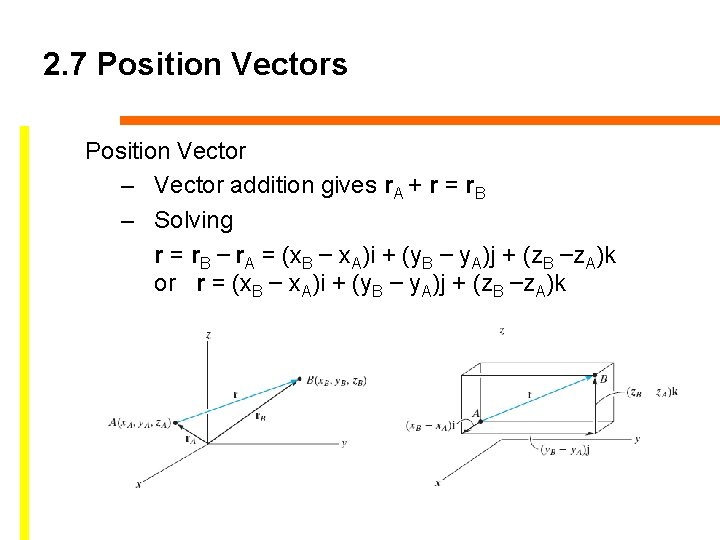2. 7 Position Vectors Position Vector – Vector addition gives r. A + r = r. B – Solving r = r. B – r. A = (x. B – x. A)i + (y. B – y. A)j + (z. B –z. A)k or r = (x. B – x. A)i + (y. B – y. A)j + (z. B –z. A)k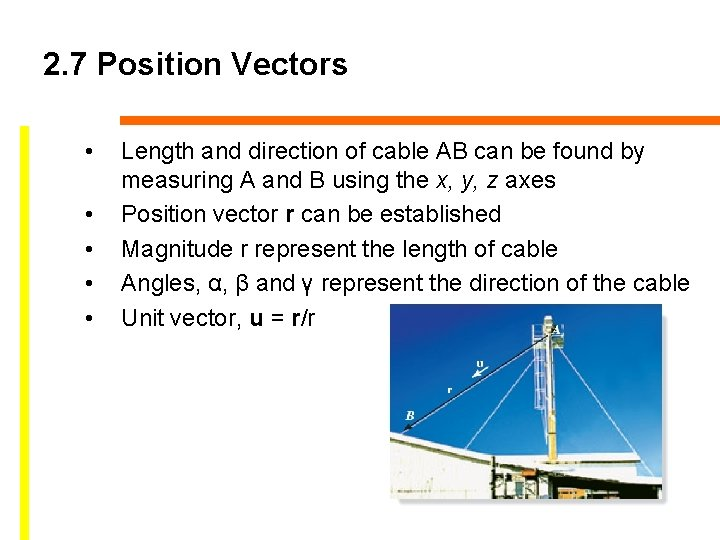2. 7 Position Vectors • • • Length and direction of cable AB can be found by measuring A and B using the x, y, z axes Position vector r can be established Magnitude r represent the length of cable Angles, α, β and γ represent the direction of the cable Unit vector, u = r/r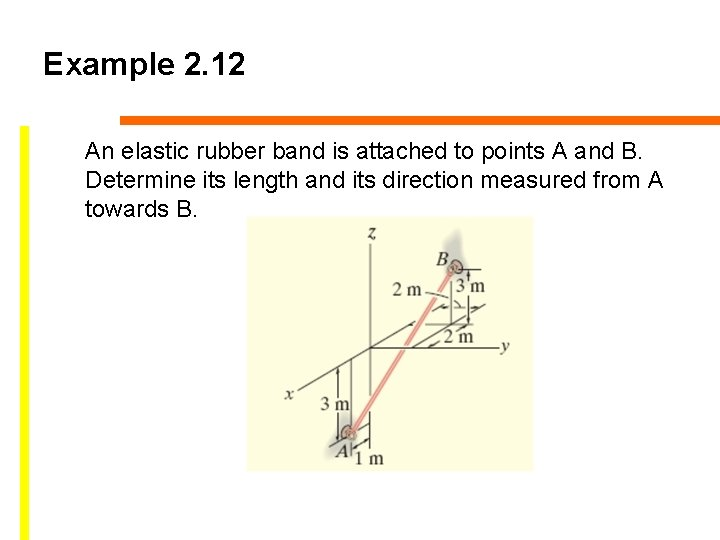Example 2. 12 An elastic rubber band is attached to points A and B. Determine its length and its direction measured from A towards B.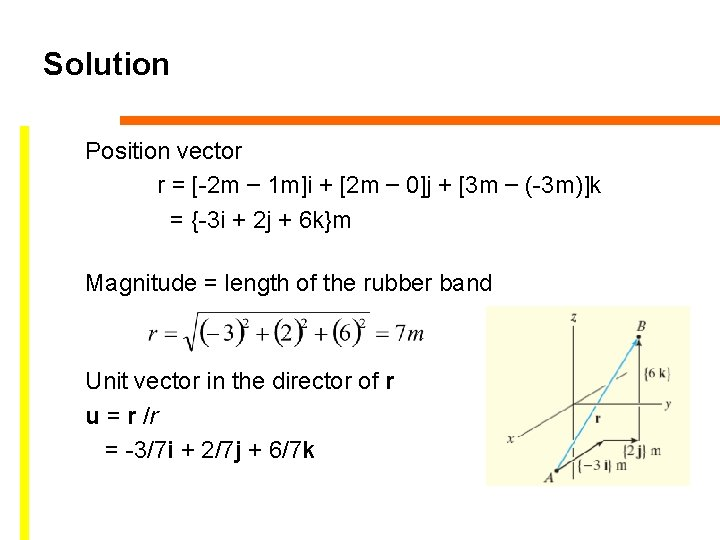Solution Position vector r = [-2 m – 1 m]i + [2 m – 0]j + [3 m – (-3 m)]k = {-3 i + 2 j + 6 k}m Magnitude = length of the rubber band Unit vector in the director of r u = r /r = -3/7 i + 2/7 j + 6/7 kSolution α = cos-1(-3/7) = 115° β = cos-1(2/7) = 73. 4° γ = cos-1(6/7) = 31. 0°2. 8 Force Vector Directed along a Line • • • In 3 D problems, direction of F is specified by 2 points, through which its line of action lies F can be formulated as a Cartesian vector F = F u = F (r/r) Note that F has units of forces (N) unlike r, with units of length (m)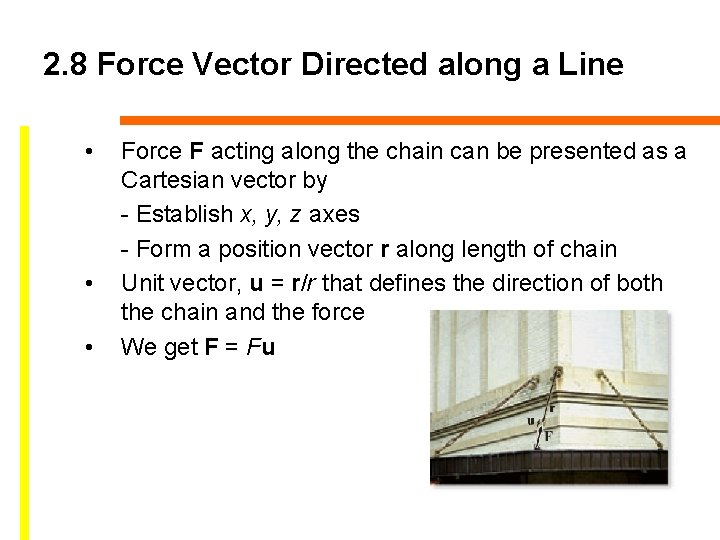2. 8 Force Vector Directed along a Line • • • Force F acting along the chain can be presented as a Cartesian vector by - Establish x, y, z axes - Form a position vector r along length of chain Unit vector, u = r/r that defines the direction of both the chain and the force We get F = FuExample 2. 13 The man pulls on the cord with a force of 350 N. Represent this force acting on the support A, as a Cartesian vector and determine its direction.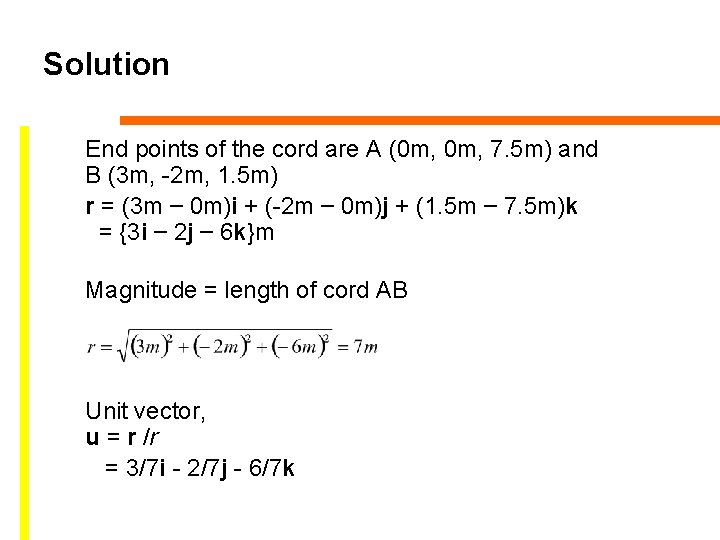Solution End points of the cord are A (0 m, 7. 5 m) and B (3 m, -2 m, 1. 5 m) r = (3 m – 0 m)i + (-2 m – 0 m)j + (1. 5 m – 7. 5 m)k = {3 i – 2 j – 6 k}m Magnitude = length of cord AB Unit vector, u = r /r = 3/7 i - 2/7 j - 6/7 kSolution Force F has a magnitude of 350 N, direction specified by u. F = Fu = 350 N(3/7 i - 2/7 j - 6/7 k) = {150 i - 100 j - 300 k} N α = cos-1(3/7) = 64. 6° β = cos-1(-2/7) = 107° γ = cos-1(-6/7) = 149°